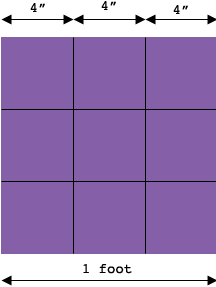SEARCH HOMEMath Central Quandaries & QueriesQuestion from Jill, how many 4" x 4" square tiles would I need for 50 square feet?Hi Jill,

$3 \times 4" = 12" = 1 \mbox{ foot}$and hence it takes $3 \times 3 = 9,$ 4 inch square tiles to make a square foot. You need to cover 50 square feet so that will take at least $9 \times 50 = 450$ tiles. Depending on the shape of the region you want to cover there may be some cutting required and hence some cutting. Hence you may need more than 450 tiles.

PennyMath Central is supported by the University of Regina and The Pacific Institute for the Mathematical Sciences.